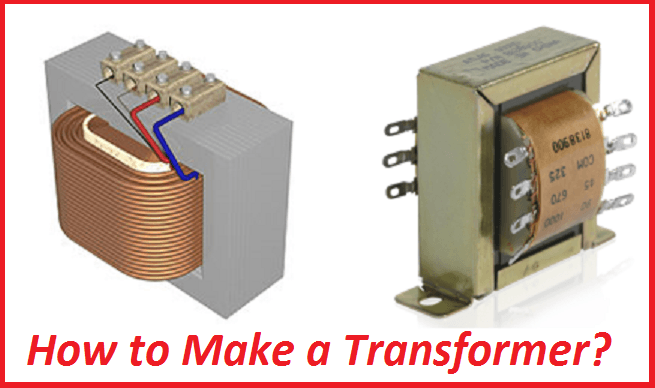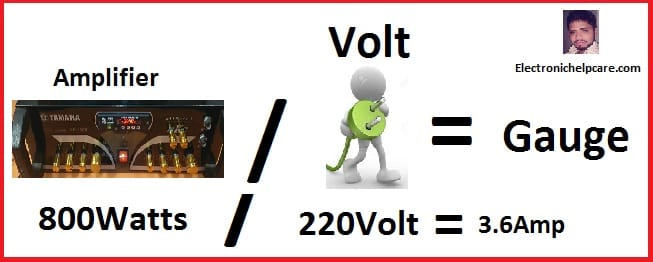# Build a Transformer or constructing transformer.

## Build a Transformer. Building or constructing transformer.

first, we need to know how to make constructing transformer? To build a transformer we need to know where we are going to use the transformer. how many Voltage, Watts and ampere need. Before all, we must know about the wire gauge and ampere. we are going to constructing transformer.

Constructing a Transformer:  How to calculate the wire ampere?Gauge     –      Ampere

7              –        44.2

8              –        33.3

9              –        26.5

10            –        21.2

11            –        16.6

12            –        13.5

13            –        10.5

14            –          8.3

15            –          6.6

16            –          5.2

17            –          4.1

18            –          3.2

19            –          2.6

20            –          2.0

21            –          1.6

22            –          1.2

23            –          1.0

24            –          0.8

25            –          0.6

26            –          0.5

27            –          0.4

28            –          0.3

29            –          0.29

30            –          0.22

How to calculate the wire ampere?

How do we know that how many amperes we need?

First, we have to decide where we are going to use this transformer.

So, How many Watts we need?

## For an example,

If we want to make 800 Watts amplifier. Then we need 800 Watts transformer.

## First for the primary binding

We know that,Watts / AC Voltage=Ampere.

So 800watts/220voltage=3.6amp

The 3.6-ampere wire we need for the primary wire

we can see the list, 18 number wire is 3.2 amperes and 17 number wire is 4.1 amperes. But we need 3.6 amperes.

We can use 17 number for good performance.

## 2nd for the secondary binding

We need 800 Watts transformer

How many Voltage and ampere do we need?

we know Voltage X Ampere = Watts.

If we Use 50 Voltage So how many Amperes we need?

How many ampere?

800/50=16 Ampere Gauge Wire.

We need 16 amperes Gauge wire.

According to our Gauge List

11 number Wire is 16.6 Amperes

So we have to use 11number gauge wire for the secondary Binding.

here have a video of the binding transformer. we can calculate the core area and also can calculate how Many turns need for binding the transformer. and how to build a transformer. if you watch the video you can learn. but this is a small transformer. don’t worry all transformer are the same rules to make.

Now let’s Go to Watch the video,

## How to constructing transformer?

transistor circuit diagram of 2sa1943 and 2sc5200

Thanks a lot to be with us. If you want another post then please visit our website.

We have another post for you. like as repairing amplifier,